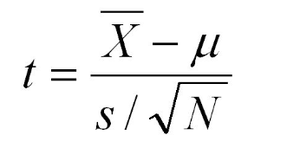# Type II Error in Lower Tail Test of Population Mean with Unknown Variance in R

• Last Updated : 02 Jun, 2022

Conventionally, In a lower-tail test, the null hypothesis states that the true population mean (μo) is greater than the hypothesized mean value (μ). We fail to reject the null hypothesis if the test statistic is greater than the critical value at the chosen significance level. In this article let us discuss the probability percentage of type II error for a lower tail test of the population mean with unknown variance.

Type II error is an error that occurs if the hypothesis test based on a random sample fails to reject the null hypothesis even when the true population mean μo is lesser than the hypothesized mean value μ.

Here the assumption is the population variance σ2  is unknown. Let s2 be the sample variance. For larger n (usually >30), the population of the following statistics of all possible samples of size n is approximately a Student t distribution with n – 1 degree of freedom (dof).

The range of sample means for a student t distribution is calculated as followstest statistic

The above formulation helps us to compute the range of sample means for which the null hypothesis will not be rejected and thereby calculate the probability of type II error.

Let us try to understand the type II error by considering a case study.

Suppose the manufacturer claims that the mean lifetime of a tyre is more than 10,000 km. Assume the actual mean tyre lifetime is 9,950 km and the sample standard deviation is 120 km. At the .05 significance level, what is the probability of having a type II error for a sample size of 30 tyres.

Code:

Let us start by computing the standard error of the mean as shown

## R

 `n = 30 ``# sample size  ` `s = 120 ``# sample standard deviation  ` `SE = s/``sqrt``(n) ` `SE ``# standard error estimate  `

Output:

`21.9089023002066`

Then compute the lower bound of sample means for which the null hypothesis μo >= 10000 would not be rejected.

## R

 `alpha = .05 ``# significance level  ` `mu0 = 10000 ``# hypothetical lower bound  ` `q = mu0 + ``qt``(alpha, df=n-1) * SE ` `q  `

Output:

`9962.77399198004`

The lower bound value denotes, that as long as the sample mean is greater than 9962 in a hypothesis test, the null hypothesis will not be rejected. Now let’s compute the probability of the sample mean being above 9962 as we know that the actual population mean is 9950. Based on this probability we can find the probability of type II error.

## R

 `mu = 9950 ``# assumed actual mean  ` `pt``((q - mu)/SE, df=n-1, lower.tail=``FALSE``)  `

Output:

`0.282183182187887`

If the tyre sample size is 30, the sample standard variance is 120 km and the actual mean tyre lifetime is 9,950 hours, then the probability of type II error for testing the null hypothesis μ ≥ 10000 at a .05 significance level is 28.21%, and the power of the hypothesis test is 71.78%.

My Personal Notes arrow_drop_up
Recommended Articles
Page :